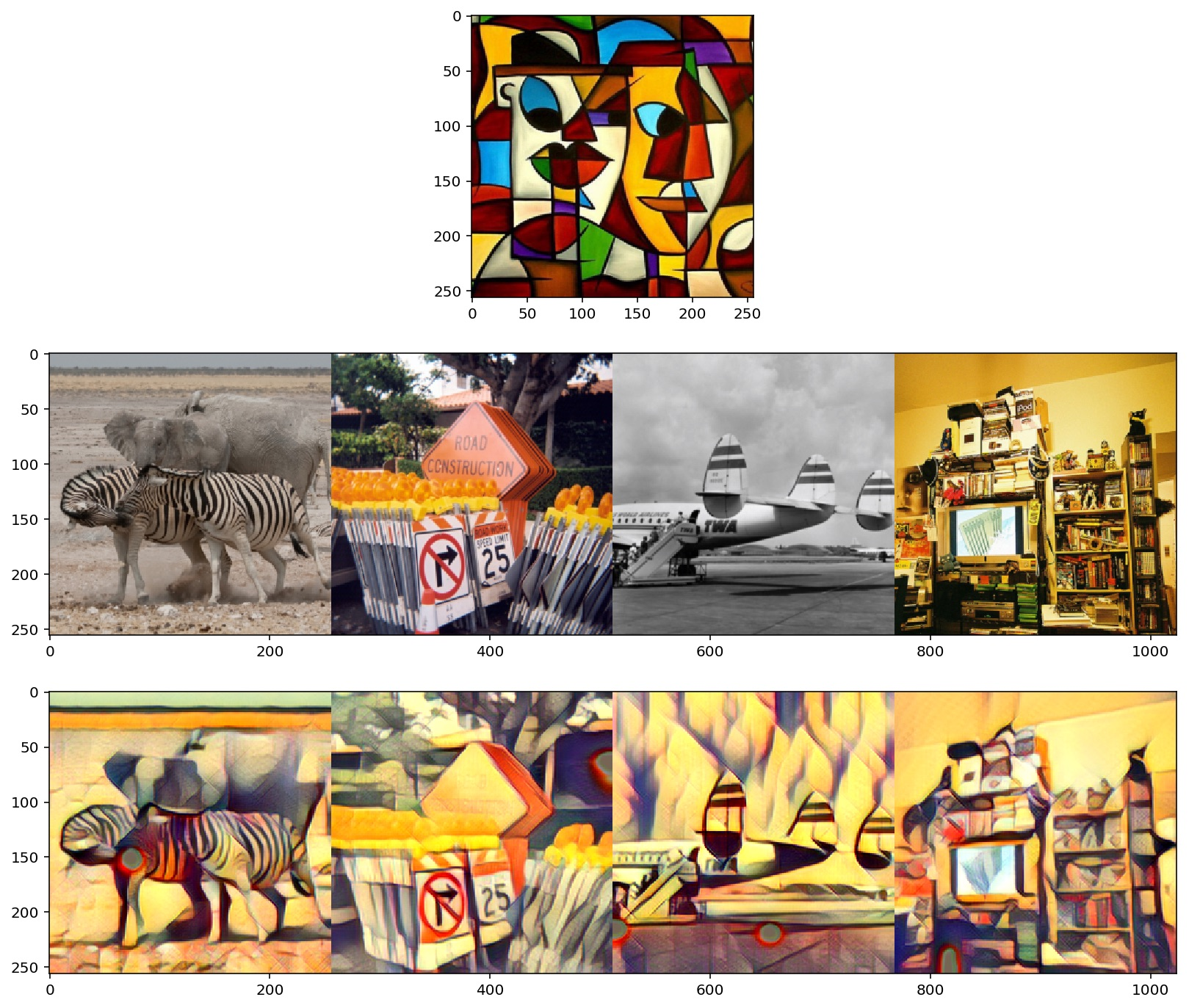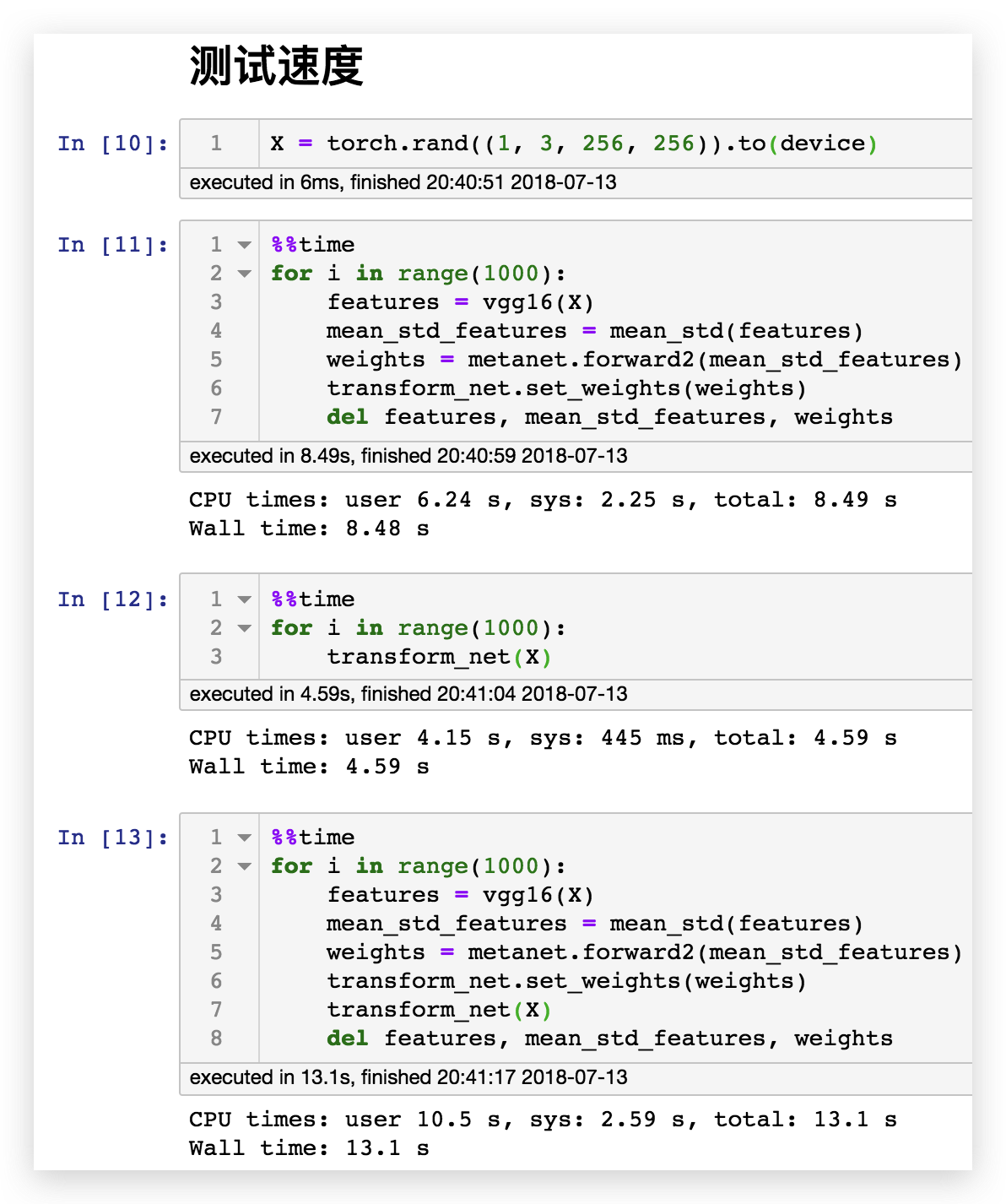# 固定风格固定内容的普通风格迁移

## VGG16

VGG16 是一个很经典的模型，它通过堆叠 3x3 的卷积层和池化层，在 ImageNet 上获得了不错的成绩。我们使用在 ImageNet 上经过预训练的 VGG16 模型可以对图像提取出有用的特征，这些特征可以帮助我们去衡量两个图像的内容差异和风格差异。

• relu1_2 [1, 64, 512, 512]
• relu2_2 [1, 128, 256, 256]
• relu3_3 [1, 256, 128, 128]
• relu4_3 [1, 512, 64, 64]

## 内容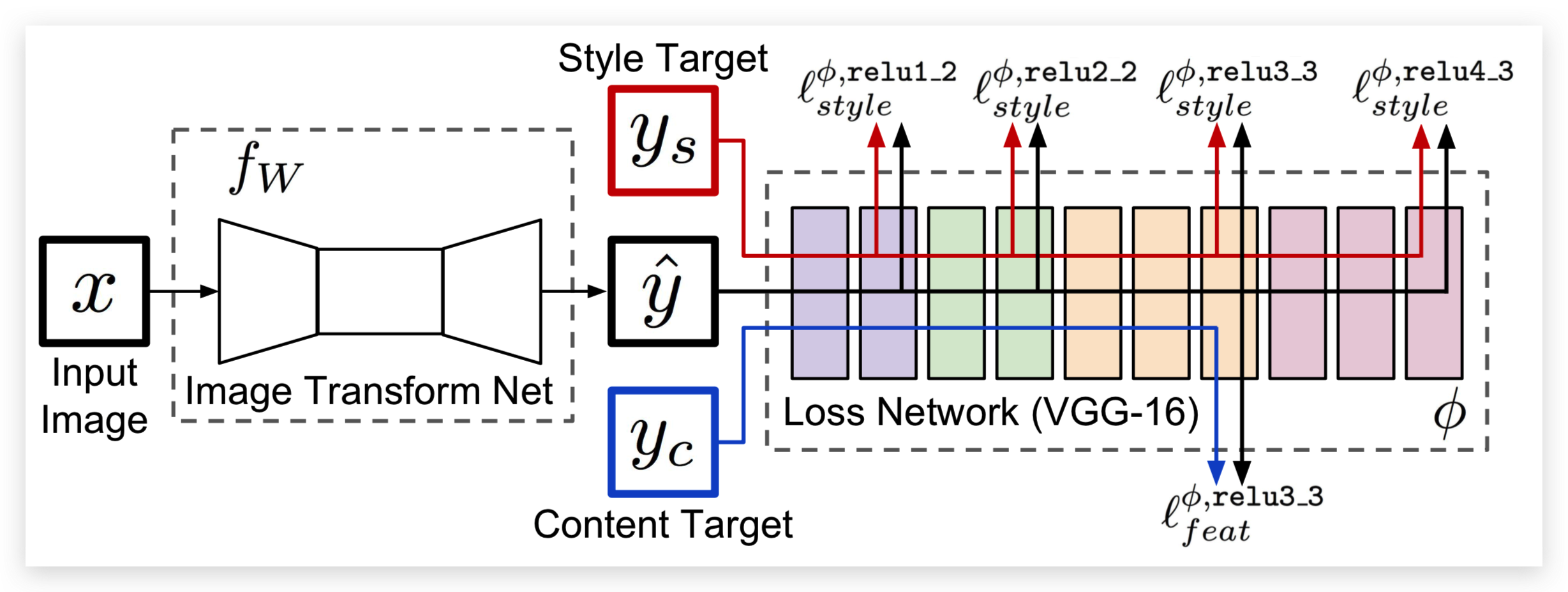$$\Large\ell^{\phi,j}_{feat}(\hat{y},y)=\frac{1}{C_jH_jW_j}||\phi_j(\hat{y})-\phi_j(y)||^2_2$$

• $\hat{y}$是输入图像（也就是生成的图像）
• $y$是内容图像
• $\phi$ 代表 VGG16
• $j$ 在这里是 $\text{relu3_3}$
• $\phi_j(x)$指的是 x 图像输入到 VGG 以后的第 j 层的特征图
• $C_j\times H_j\times W_j$是第 j 层输出的特征图的尺寸

## 风格

### Gram 矩阵

$$\Large{G^\phi_j(x)_{c,c’}=\frac{1}{C_jH_jW_j} \sum_{h=1}^{H_j} \sum_{w=1}^{W_j} \phi_j(x)_{h,w,c}\phi_j(x)_{h,w,c’}}$$

• $\hat{y}$是输入图像（也就是生成的图像）
• $y$是风格图像
• $C_j\times H_j\times W_j$是第 j 层输出的特征图的尺寸。
• $G^\phi_j(x)$指的是 x 图像的第 j 层特征图对应的 Gram 矩阵，比如 64 个卷积核对应的卷积层输出的特征图的 Gram 矩阵的尺寸是 $(64, 64)$。
• $G^\phi_j(x)_{c,c’}$ 指的是 Gram 矩阵第 $(c, c’)$ 坐标对应的值。
• $\phi_j(x)$指的是 x 图像输入到 VGG 以后的第 j 层的特征图，$\phi_j(x)_{h,w,c}$ 指的是特征图 $(h,w,c)$坐标对应的值。

Gram 矩阵的计算方法其实很简单，Gram 矩阵的 $(c, c’)$ 坐标对应的值，就是特征图的第 $c$ 张和第 $c’$ 张图对应元素相乘，然后全部加起来并且除以 $C_j\times H_j\times W_j$ 的结果。根据公式我们可以很容易推断出 Gram 矩阵是对称矩阵。

https://github.com/pytorch/examples/blob/0.4/fast_neural_style/neural_style/utils.py#L21-L26

• relu1_2 [1, 64, 512, 512]，gram [1, 64, 64]
• relu2_2 [1, 128, 256, 256]，gram [1, 128, 128]
• relu3_3 [1, 256, 128, 128]，gram [1, 256, 256]
• relu4_3 [1, 512, 64, 64]，gram [1, 512, 512]

### 风格损失

$$\Large\ell^{\phi,j}_{style}(\hat{y},y)=||G^\phi_j(\hat{y})-G^\phi_j(y)||^2_F$$

• $\hat{y}$是输入图像（也就是生成的图像）
• $y$是风格图像
• $G^\phi_j(x)$指的是 x 图像的第 j 层特征图对应的 Gram 矩阵

## 效果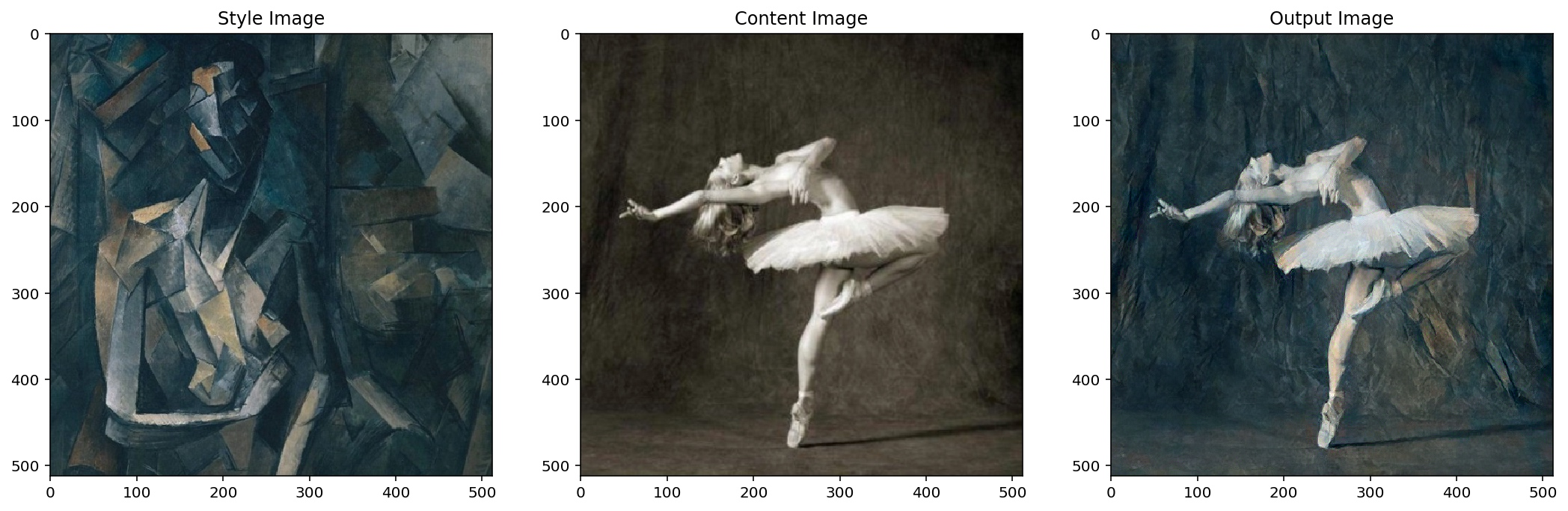# 固定风格任意内容的快速风格迁移

## 模型

• 降维，三层卷积层，逐渐提升通道数为128，并且通过 stride 把特征图的宽高缩小为原来的八分之一
• 5个 ResidualBlock 堆叠
• 升维，三层卷积层，逐渐降低通道数为3，并且通过 nn.Upsample 把特征图的宽高还原为原来的大小

### TransformNet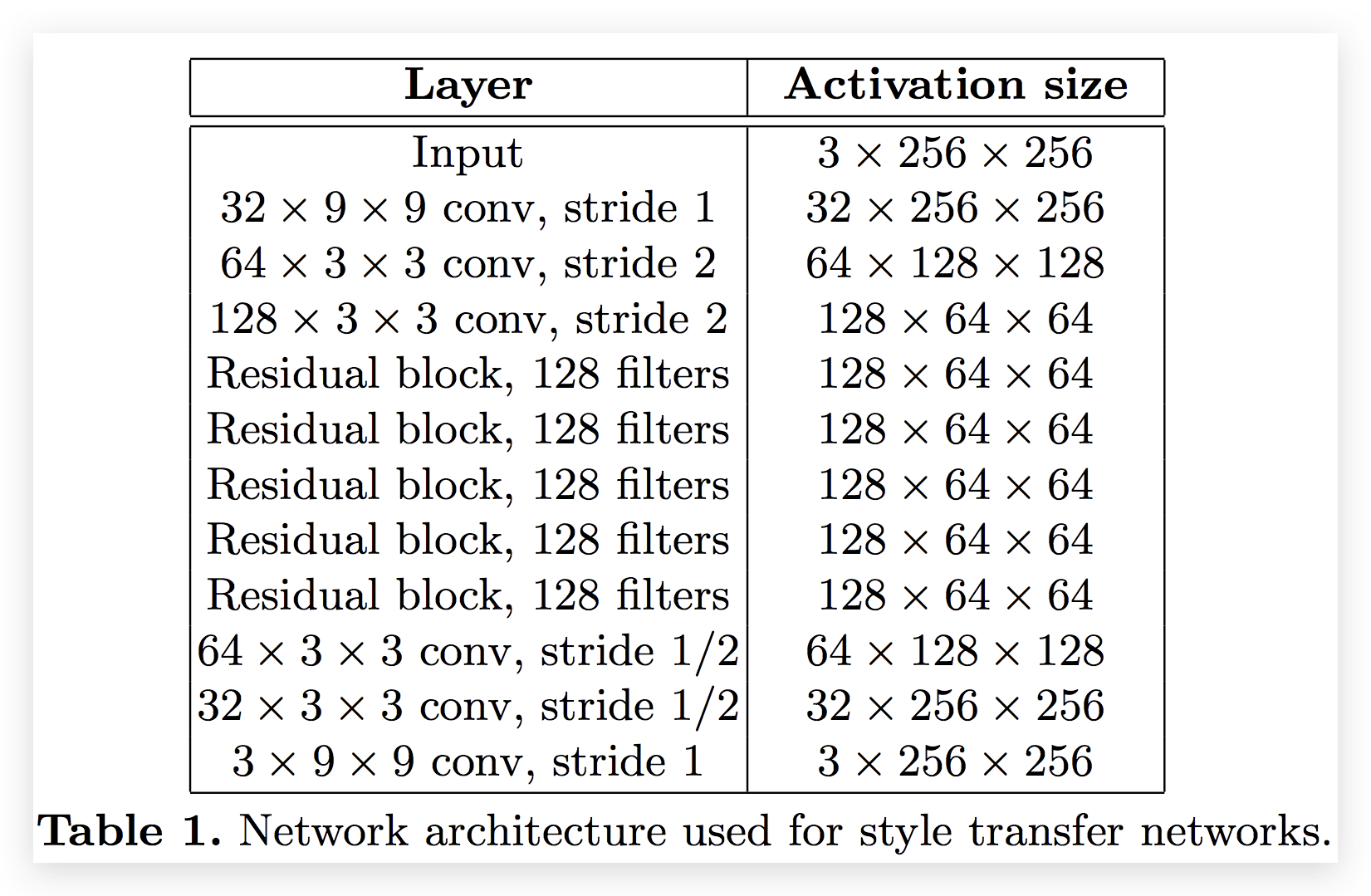## 数据

We resize each of the 80k training images to 256 × 256 and train our networks with a batch size of 4 for 40,000 iterations, giving roughly two epochs over the training data.

## 训练

### 超参数

We use Adam  with a learning rate of 1 × 10−3.

The output images are regularized with total variation regularization with a strength of between $1\times10^{-6}$ and $1\times10^{-4}$, chosen via cross-validation per style target.

tv_weight 感觉没有太大变化，所以按论文中给出的参考设置了 1e-6。

train our networks with a batch size of 4 for 40,000 iterations

batch_size 按论文设置为了4。

### TotalVariation

Total Variation Regularization. To encourage spatial smoothness in the output image $\hat{y}$, we follow prior work on feature inversion [6,20] and super- resolution [48,49] and make use of total variation regularizer $\ell_{TV}(\hat{y})$.

$$\Large{V_\text{aniso}(y)=\sum_{i,j}|y_{i+1,j}-y_{i,j}|+|y_{i,j+1}-y_{i,j}|}$$

## 效果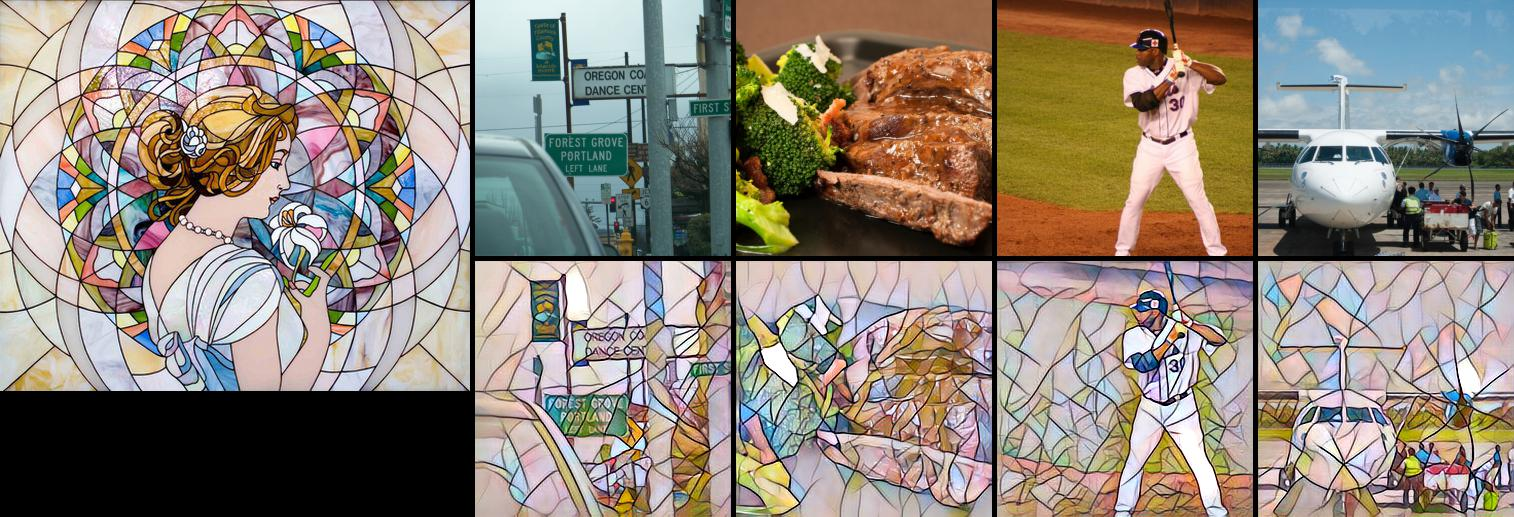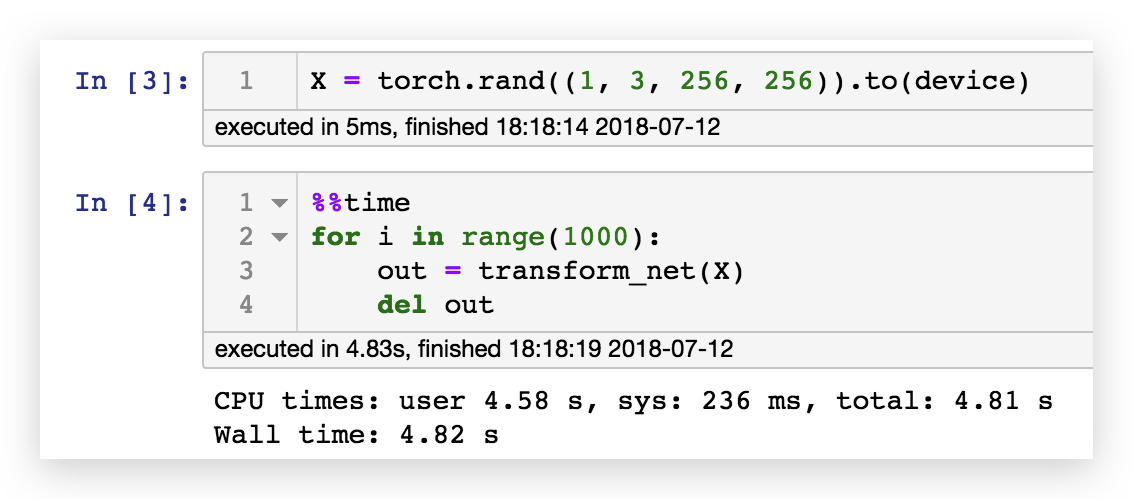# 任意风格任意内容的极速风格迁移

## 情况1

$$\large{\min_I\left(\lambda_c||\mathbf{CP}(I;w_f)-\mathbf{CP}(I_c;w_f)||^2_2+ \lambda_s||\mathbf{SP}(I;w_f)-\mathbf{SP}(I_s;w_f)||^2_2\right)}$$

• $\mathbf{CP}$ 是内容损失函数
• $\mathbf{SP}$ 是风格损失函数
• $\lambda_c$ 是内容权重
• $\lambda_s$ 是风格权重
• $w_f$ 是VGG16的固定权值
• $I_s$ 是风格图像
• $I_c$ 是内容图像
• $I$ 是输入图像

## 情况2

$$\large{\min_w\sum_{I_c}\left(\lambda_c||\mathbf{CP}(I_w;w_f)-\mathbf{CP}(I_c;w_f)||^2_2+ \lambda_s||\mathbf{SP}(I_w;w_f)-\mathbf{SP}(I_s;w_f)||^2_2\right)}$$

• $I_w$ 是生成图像，$I_w=\mathcal{N}(I_c;w)$，$\mathcal{N}$ 是图像转换网络

## 情况3

$$\large{\min_\theta\sum_{I_c,I_s}\left(\lambda_c||\mathbf{CP}(I_{w_\theta};w_f)-\mathbf{CP}(I_c;w_f)||^2_2+ \lambda_s||\mathbf{SP}(I_{w_\theta};w_f)-\mathbf{SP}(I_s;w_f)||^2_2\right)}$$

• $\theta$ 是 $Meta\mathcal{N}$ 的权值
• $w_\theta$ 是转换网络的权值，$w_\theta=Meta\mathcal{N}(I_s;\theta)$，所以我们可以说转换网络的权值是 MetaNet 通过风格图像生成的。
• $I_{w_\theta}$ 是转换网络生成的图像，$I_{w_\theta}=\mathcal{N}(I_c;w_\theta)$

## 转换网络（TransformNet）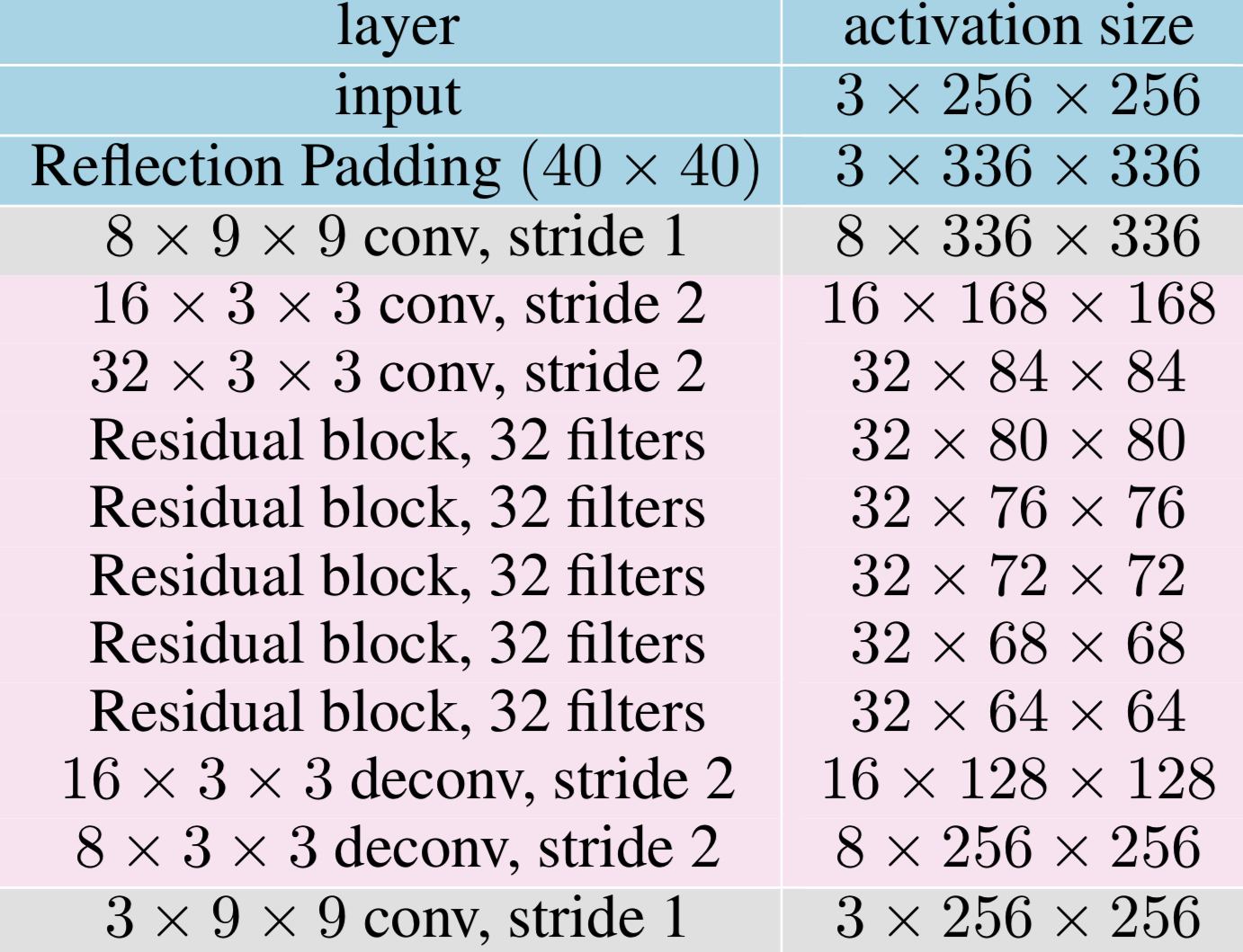### MyConv2D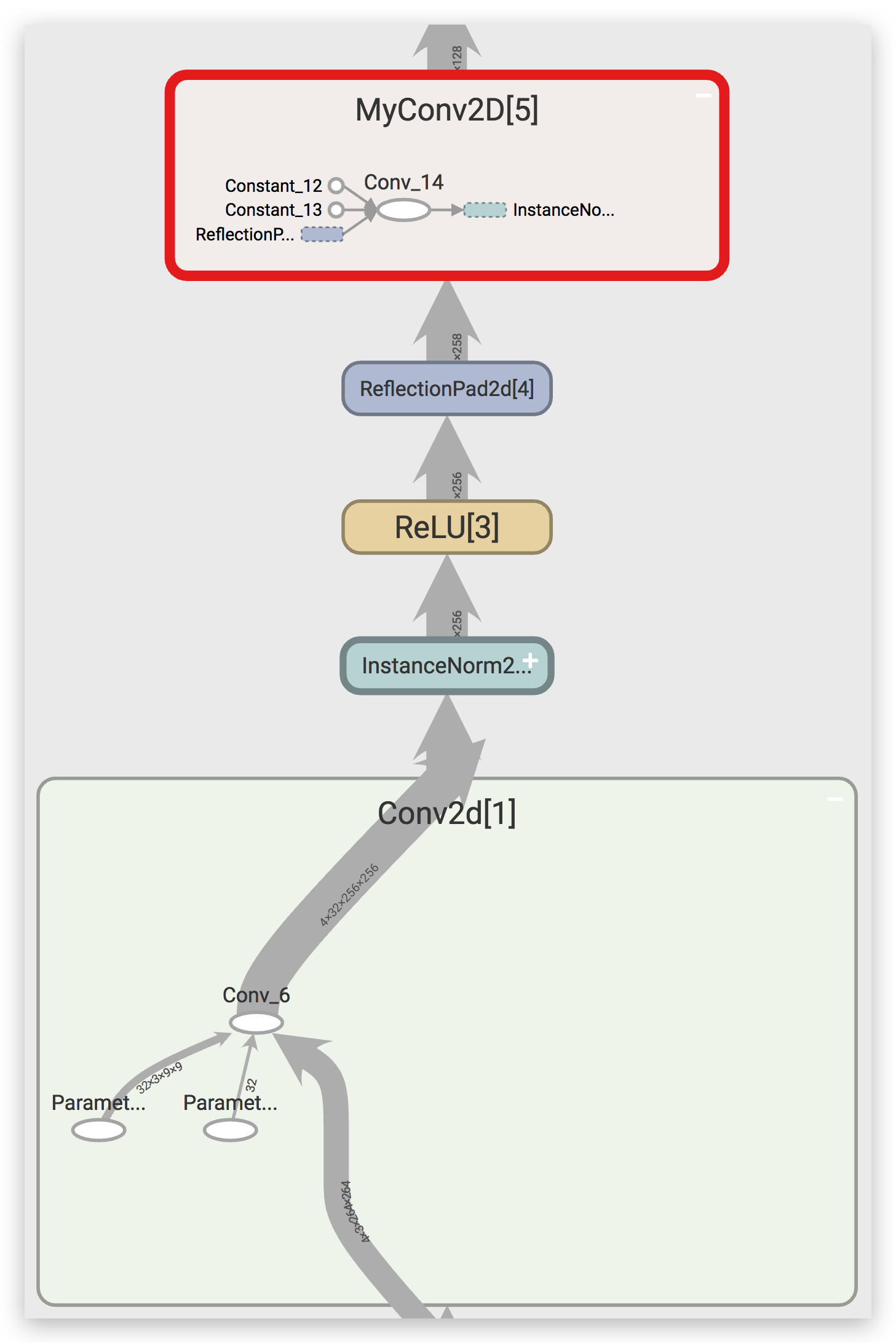### ConvLayer

• 权值是是可训练的参数
• 权值由 MetaNet 给出

### TransformNet

TransformNet(32) 每一层对应的权重数量如下：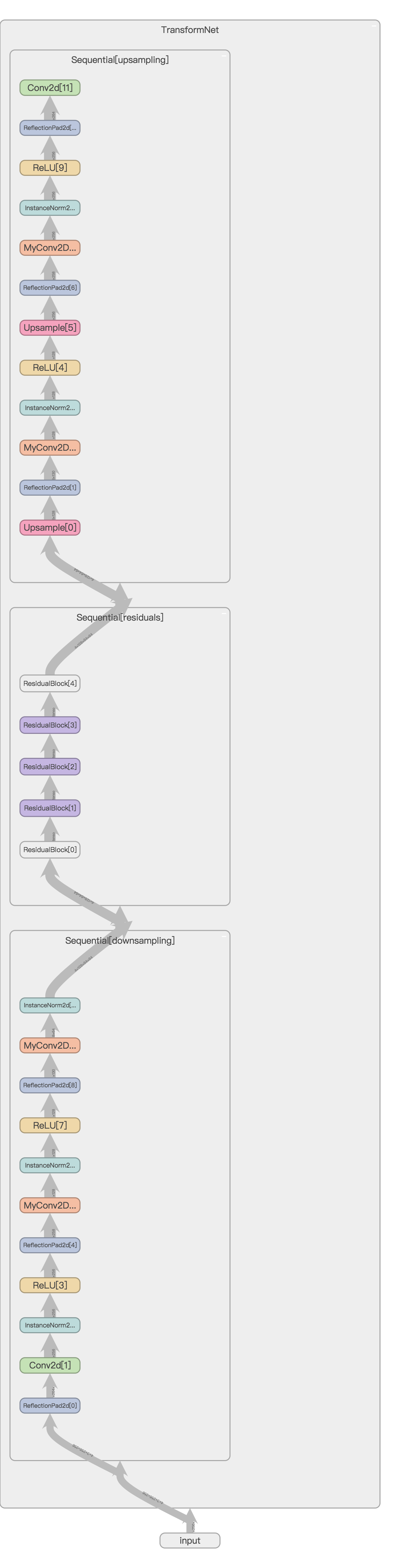## MetaNet

We compute the mean and stand deviations of two feature maps of the style image and the transferred image as style features.

The dimension of hidden vector is 1792 without specification. The hidden features are connected with the filters of each conv layer of the network in a group manner to decrease the parameter size, which means a 128 dimensional hidden vector for each conv layer.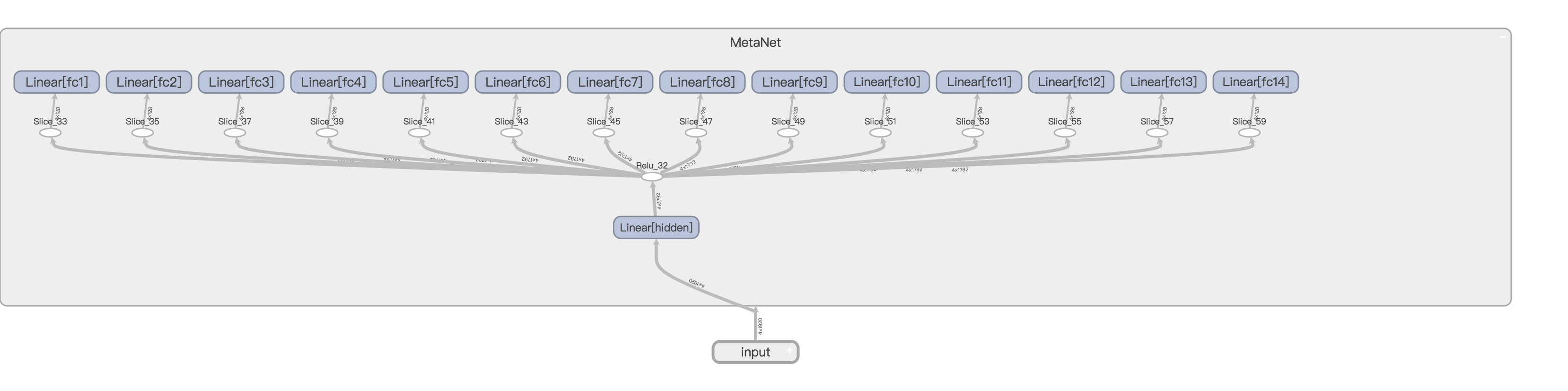## 数据

There are about 120k images in MS- COCO trainval set and about 80k images in the test set of WikiArt.

During training, each content image or style image is resized to keep the smallest dimension in the range [256, 480], and randomly cropped regions of size 256 × 256.

## 训练

### 超参数

The weight of content loss is 1 while the weight of style loss is 250.

We use Adam (Kingma and Ba 2014) with fixed learning rate 0.001 for 600k iterations without weight decay.

The transferred images are regularized with total variations loss with a strength of 10.

The batch size of content images is 8 and the meta network is trained for 20 iterations before changing the style image.

### 代码

1. 如果内容图像是纯色的，那么权值会直接 nan，原因不明，为了避免这个问题，需要检查纯色，然后 continue 来避免 nan。
2. 权值会逐渐增大，目前没有比较好的解决方案。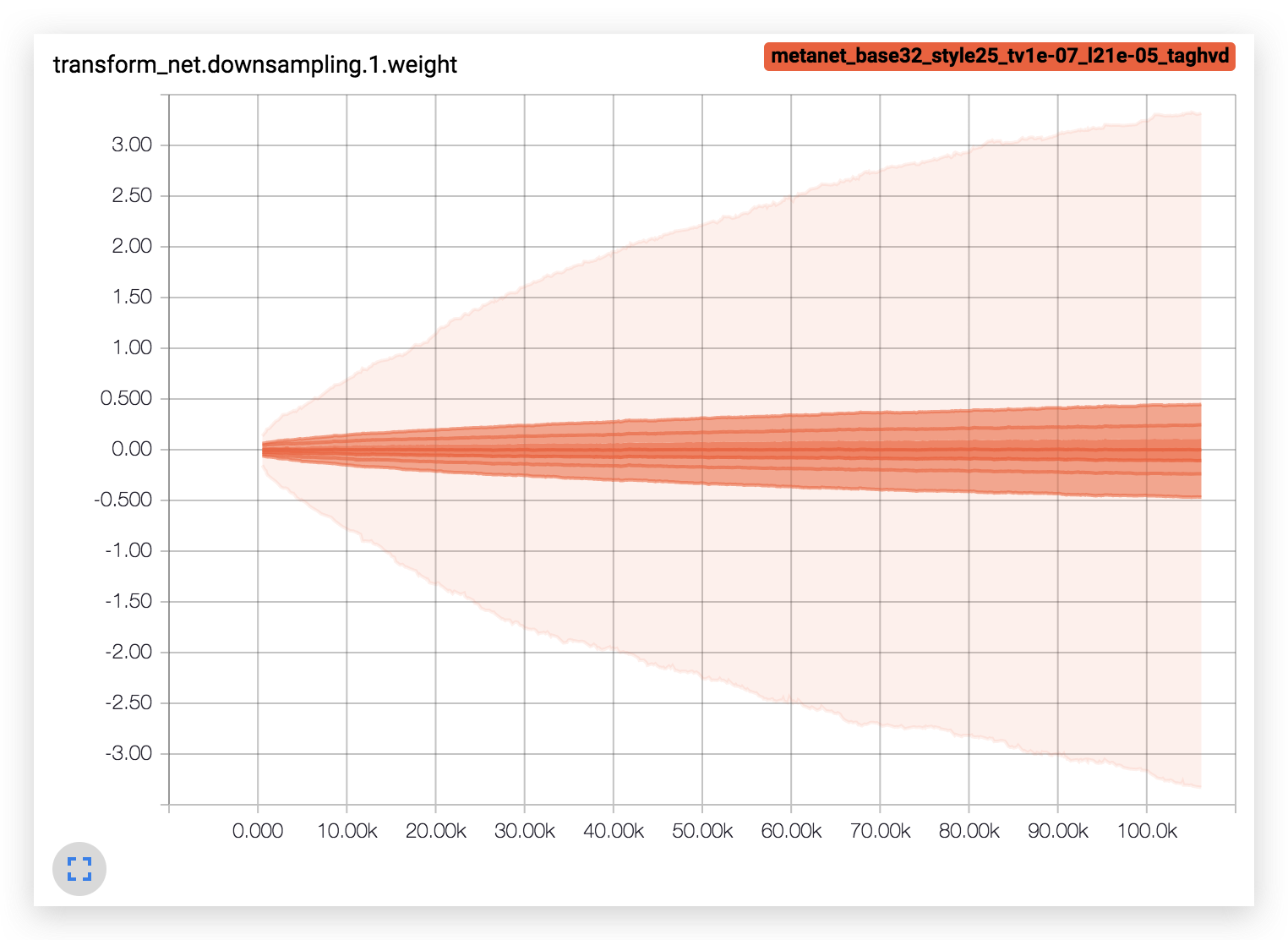## 效果